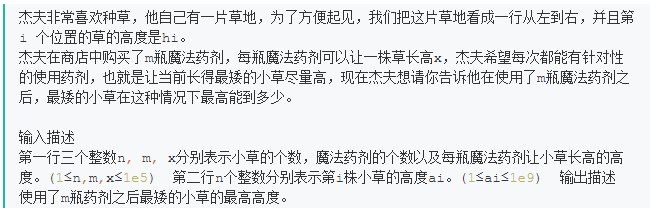# 广联达21届提前批0729笔试（截图是从牛客帖子上找的，不是我考试时截的）

#include<iostream>
#include<algorithm>
#include<vector>
using namespace std;
int main() {
int n, m, x;
cin >> n >> m >> x;
vector<int>a(n, 0);
for (int i = 0; i < n; i++) {
cin >> a[i];
}
sort(a.begin(), a.end());
int min0 = a;
min0 = a + x;
a += x;
for (int i = 1; i < m; i++) {
sort(a.begin(), a.end());
int curMin = a;
if (curMin == min0) {
min0 += x;
}
a + x;
}
cout << min0 << endl;
return 0;
}


（待完善。。。）

07-24609
08-01196309-268026
05-031715
12-01
08-303515
08-26334
08-19160